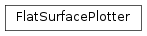# mvpa2.misc.plot.flat_surf¶

Plot flat maps of cortical surfaces.

WiPFunctions

 `curvature_from_any`(c) Reads curvature `flat_surface2grid_mask`(surface, min_nsteps, ...) Computes a mask and corresponding coordinates from a flat surface `flat_surface2xy`(surface, max_deformation) Returns a tuple with x and y coordinates of a flat surface `flat_surface_curvature2rgba`(curvature) Computes an RGBA colormap in black and white, based on curvature `flat_surface_data2rgba`(data[, range_, ...]) Computes an RGBA colormap for surface data `griddata`(x, y, z, xi, yi[, interp]) Interpolates from a nonuniformly spaced grid to some other grid. `nanmax_wrapper`(xs) `nanmin_wrapper`(xs) `unstructured_xy2grid_xy_vectors`(x, y, min_nsteps) From unstructured x and y values, return lists of x and y coordinates `vector_alignment_find_rotation`(x, y) Find rotation matrix to align one vector with another

Classes

 `Dataset`(samples[, sa, fa, a]) Generic storage class for datasets with multiple attributes. `FlatSurfacePlotter`(surface[, curvature, ...]) Plots data on a flat surface